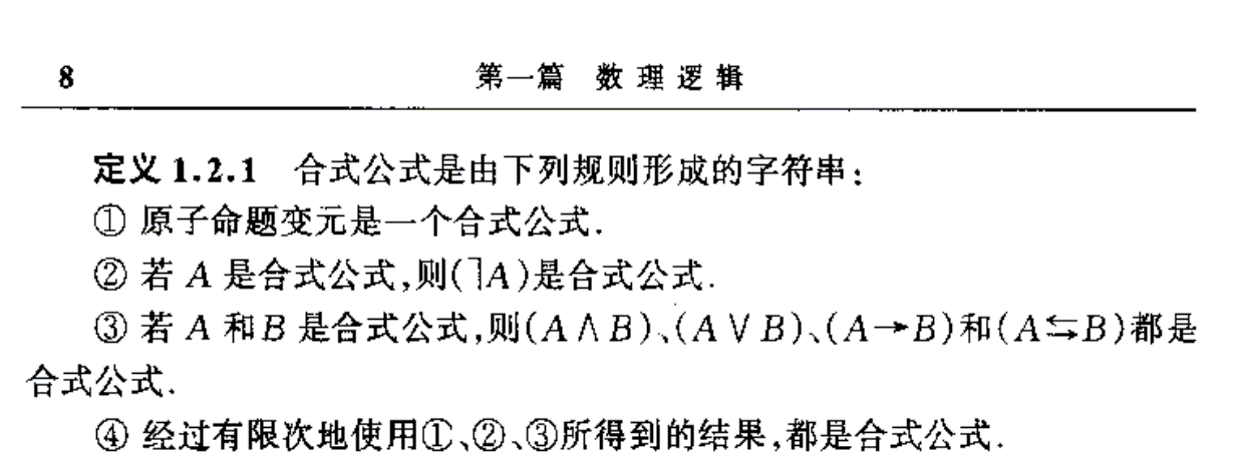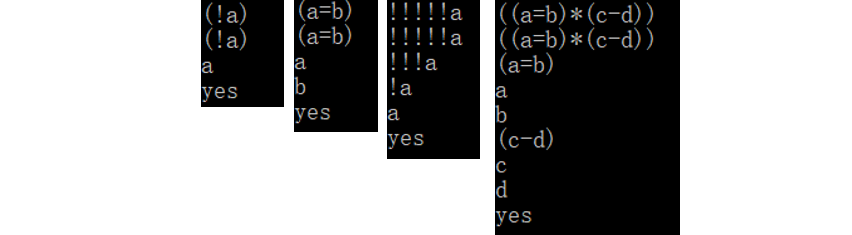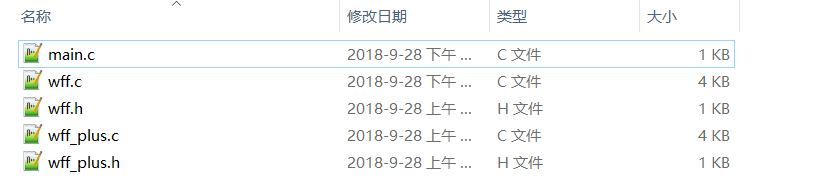• 【应用C】C语言实现离散数学合式公式的递归判断-附件资源
•C语言
• 构造任意合式公式的真值表构造任意合式公式的真值表#include "stdio.h"#include"thesis.h"int main(){Thesis a;char x='1';int i=0,N;cout<while(int(x)!=48){cin>>x;if(i>19){cout<if(x!='0'){a...

构造任意合式公式的真值表

构造任意合式公式的真值表

#include "stdio.h"

#include"thesis.h"

int main()

{

Thesis a;

char x='1';

int i=0,N;

cout<

while(int(x)!=48)

{

cin>>x;

if(i>19)

{cout<

if(x!='0')

{

a[i].inname(x);

i++;

}

}

N=i;

int M;

M=N;

string A;

cout<

cin>>A;

cout<

for(int j=0;j

cout<

cout<

assignment(A,N,M,&a);

system("pause");

return 0;

}

#include"thesis.h"

#ifndef THESIS_H

#define THESIS_H

#include

#include

#include

using namespace std;

class Thesis //命题类

{

int value;char name; //value:命题的真值(0/1) name：命题名

public:

Thesis(){value=2;name='A';};

friend Thesis operator !(Thesis &q)

{q.invalue(1-q.getvalue()); return q;} //重载逻辑运算符

friend Thesis operator &(Thesis &p,Thesis &q)

{p.invalue((p.getvalue()+q.getvalue())/2); return p;}

friend Thesis operator |(Thesis &p,Thesis &q)

{if(p.getvalue()+q.getvalue()>0) p.invalue(1);

else p.invalue(0);

return p;}

friend Thesis operator >(Thesis &p,Thesis &q)

{if(p.getvalue()==1&&q.getvalue()==0)

p.invalue(0);

else p.invalue(1);

return p;}

friend Thesis operator

{if(p.getvalue()==q.getvalue()) p.invalue(1);

else p.invalue(0);

return p;}

void invalue(int x){value=x;} //输入value

void inname(char x){name=x;} //输入name

int getvalue(){return value;} //获取真值

int getname(){return name;} //获取命题名

};

void assignment(string A,int N,int M,Thesis a[]); //声明函数

int bds(string A,int N,Thesis a[]);

int run(string A,int &i,int L,int N,Thesis a[]);

void assignment(string A,int N,int M,Thesis a[])//命题赋值并计算真值

{

for(int j=0;j<2;j++)

{

a[N-1].invalue(j);

if(N>1)

{

assignment(A,N-1,M,&a);

}

else

{

for(int i=0;i

{

cout<

展开全文• 构造任意合式公式的真值表A)功能给出任意变元的合式公式，要求构造该合式公式的真值表。B)基本思想我们仍然用数值变量来表示命题变元。合式公式的表示及求真值的方法采用1.2 中所采用的方法，并在程序计算之前将转换...构造任意合式公式的真值表

A)功能

给出任意变元的合式公式，要求构造该合式公式的真值表。

B)基本思想

我们仍然用数值变量来表示命题变元。合式公式的表示及求真值的方法采用1.2   中所采用的方法，并在程序计算之前将转换后的合式公式输入到本程序200语句中条件位置上。另外，我们使用一维数组A(N)来表示合式公式中所出现的n个命题变元。例如合式公式：

(P∨Q)∧((P∨R)   ∨S)应表示成以下语句：

200   IF   NOT   (A(1)=1   OR   A(2)=1)AND   ((A(1)=1   OR   A(3)=1)   OR   A(4)=1)   THEN     Z=1   ELSE   Z=0

其中，一维数组A(N)除了表示n   个命题变元，它还是一个二进制加法器的模拟器，每当在这个模拟器中产生一个二进制数时，就相当于给各命题变元产生了一组真值指派。其中数值1表示真值真，而0表示值假。

C)算法

(1) 将二进制加法器模拟器A(N)赋初值。0   ai   (i=1,2,….,n)。

(2) 计算模拟器中所对应的一组真值指派下合式公式的真值(200语句)。

(3) 输出真值表中对应于模拟器所给出的一组真值指派及这组真值指派所对应的一行真值。

(4) 在模拟器A(N)，模拟的产生下一个二进制数值。

(5) 若A(N)中的数值等于2n,则结束，否则转(2)。

◆◆

评论读取中....

请登录后再发表评论!

◆◆

修改失败，请稍后尝试

展开全文• 一、判断合式公式 输入：（否定 ！，合取 & ，析取 | ，条件 - ，双条件 =）约定 A-B为A->B 由书本定理1-3.1；制定三个规则： 规则1：将原子命题全部转换为字符1； 规则2：将!1、(1)替换为字符1； 规则3：将1...

（完整代码在文末）

一、判断合式公式

输入：（否定 ！，合取 & ，析取 | ，条件 - ，双条件 =）约定 A-B为A->B
由书本定理1-3.1；制定三个规则：
规则1：将原子命题全部转换为字符1；
规则2：将!1、(1)替换为字符1；
规则3：将1|1、1&1、1-1、1=1替换为字符1。

• 定义替换函数rps（string &str,string ss,string sd,intrunum）//用"sd"替换"str"中的"ss"，用到规则runum
• 利用while循环与规则依次替换，最终如果得到字符‘1’则为合式公式，继续打印真值表、求主合取、主席取范式；否则不是合式公式，继续输入
int rps(string &str,string ss,string sd,int runum)//用"sd"替换"str"中的"ss"
{
int i=str.length(),p;
while(p=str.find(ss)+1)
str.replace(p-1, ss.length(), sd);
//if(str.length()!=i)
//cout<<"使用规则"<<runum<<"后："<<str<<endl;
return str.length()-i;
}
bool judge(string &str)//判断是否为合式公式
{
//规则1：将原子命题全部转换为字符1
//规则2：将!1、(1)替换为字符1
//规则3：将1|1、1&1、1-1、1=1替换为字符1
string strtmp=str;
for(int i=0; i<str.length(); i++)//使用规则1
if(isalpha(str[i]))
str[i]='1';
//cout<<"使用规则1后："<<str<<endl;
while(rps(str,"!1","1",2)||rps(str,"(1)","1",2)||rps(str,"1&1","1",3)||rps(str,"1|1","1",3)||rps(str,"1-1","1",3)||rps(str,"1=1","1",3));//使用规则2、3
if(str=="1")
{
cout<<strtmp<<"是合式公式"<<endl;
return true;
}
else
{
cout<<strtmp<<"不是合式公式"<<endl;
return false;
}
}

二、打印真值表

• 将合式公式中原子命题建立到set容器中
set<char>sh;//集合统计原子命题
for(int i=0;i<str.size();i++)
if(isalpha(str[i]))sh.insert(str[i]);

• 将合式公式前缀表达式转化为后缀表达式，便于判断合式公式真假
原理

1.初始化两个栈，存储中间结果的栈s1、联结词栈s2
2.从左至右扫描合式公式
3.遇到原子命题是将其压入s1
4.遇到联结词时，将其与s2栈顶元素比较
（1）若s2为空，或s2栈顶为左括号‘（’，则将联结词压入s2
（2）若较s2栈顶联结词高级则将联结词压入s2
（3）若较s2栈顶联结词低级或平级则将s2栈顶元素弹出压入s1再与新栈顶元素比较（重复4（1））
5.遇到括号时，左括号‘（’直接压入s2；遇右括号‘）’将s2中左括号前面的元素依次弹出压入s1，并将左括号出栈
6.重复步骤2-5，直到合式公式最右端
7.将s2中剩余元素依次弹出压入s1中，最后将s1中元素依次弹出存储在s3中，得到最终的后缀表达式

注意：每输入一个合式公式应清空栈

void turn(string &s)//中缀表达式转化为后缀表达式
{
stack<char> s1,s2;
for(int i=0;i<s.size();i++)//遍历字符串
{
char p=s[i];
if(isalpha(p))s1.push(p);//原子命题直接压入s1
else if(p=='(')s2.push(p);//左括号直接压入s2
else if(p==')')
{
while(!s2.empty()&&s2.top()!='(')//遇右括号将s2中左括号前面的元素依次弹出压入s1
{
s1.push(s2.top());
s2.pop();
}
s2.pop();//将左括号出栈
}
else//遇到联结词则与s2栈顶元素比较
{
if(s2.empty())s2.push(p);//栈空直接入栈
else if(s2.top()=='(')s2.push(p);//栈顶为左括号直接入栈
else if(sx.find(p)<sx.find(s2.top()))s2.push(p);//较高级则直接入栈
else
{
while(!s2.empty()&&s2.top()!='('&&sx.find(p)>=sx.find(s2.top()))//较低级或同级则将s2栈顶元素弹出压入s1再与新栈顶元素比较
{
s1.push(s2.top());
s2.pop();
}
s2.push(p);
}
}
}
while(!s2.empty())//将s2中剩余元素压入s1
{
s1.push(s2.top());
s2.pop();
}
while(!s3.empty())s3.pop();//清空s3
while(!s1.empty())//将后缀表达式存储在栈s3中
{
s3.push(s1.top());
s1.pop();
}
}

• 命题的指派

定义指派函数

void appoint(int num)
for(set<char>::iterator it=sh.begin();it!=sh.end();it++)//遍历原子命题
{
if(num%2)ch[*it]=true;
else ch[*it]=false;
num/=2;
}

利用num转化为二进制对原子命题赋值true or false

• 判断合式公式真假

定义五个运算函数

bool Fouding(bool a)//否定运算
{
if(a)return false;
else return true;
}
bool And(bool a,bool b)//合取
{
if(!a||!b)return false;
else return true;
}
bool Or(bool a,bool b)//析取
{
if(a||b)return true;
else return false;
}
bool One(bool a,bool b)//条件
{
if(a&&!b)return false;
else return true;
}
bool Two(bool a,bool b)//双条件
{
if(a==b)return true;
else return false;
}

定义后缀表达式求值函数

bool value()//后缀表达式求值
{
ch['T']=true;//定义map中T为true，F为false
ch['F']=false;
stack <char> c,s;
s=s3;//复制后缀表达式
while(!s.empty())//计算后缀表达式
{
char p=s.top();//取栈顶元素
s.pop();
if(isalpha(p))c.push(p);//原子命题直接压入c
else if(p=='!')//联结词!处理
{
char t=c.top();
c.pop();
Fouding(ch[t]) ? c.push('T'):c.push('F');
}
else //其他联结词处理
{
char ph;//定义入栈元素
char t1=c.top();//取两栈顶元素
c.pop();
char t2=c.top();
c.pop();
switch(p)
{
case '&':And(ch[t2],ch[t1]) ? ph='T':ph='F';break;
case '|':Or(ch[t2],ch[t1])  ? ph='T':ph='F';break;
case '-':One(ch[t2],ch[t1]) ? ph='T':ph='F';break;
case '=':Two(ch[t2],ch[t1]) ? ph='T':ph='F';break;
}
c.push(ph);
}
}
if(c.top()=='T')  return true;
else return false;
}

原理：

定义map映射中ch[‘T’]=true; ch[‘F’]=false;
1.建立栈c存储中间结果，栈s复制后缀表达式
2.将s中元素依次出栈扫描 若为原子命题则直接压入栈c 若为联结词‘！’则栈c出栈一个元素进行否定运算并将结果压入栈c 若为其他联结词，则栈c出栈两个元素进行运算，并将结果压入栈c 最终结果栈c中为‘T’则合式公式为真，否则为假
5.依次遍历，打印真值表

三、求主合取、主析取范式

• 定义结构体
struct node
{
string strl;
}Min,Max;//Min存储小项，Max存储大项
int l1,l2;//l1表示小项个数，l2表示大项个数

对每个合式公式，应清空字符串stl，l1、l2置零
定义求小项函数void small()，求大项函数void big()

void small()//求小项
{
Min[l1].strl.clear();//清空字符串
Min[l1].strl+='(';
int num=0;
for(set<char>::iterator it=sh.begin();it!=sh.end();it++,num++)//遍历原子命题
{
if(!ch[*it])Min[l1].strl+='!';//0 --> ！
Min[l1].strl+=*it;
if(num<sh.size()-1) Min[l1].strl+='&';
}
Min[l1].strl+=')';
l1++;//小项个数加一
}
void big()//求大项
{
Max[l2].strl.clear();//清空字符串
Max[l2].strl+='(';
int num=0;
for(set<char>::iterator it=sh.begin();it!=sh.end();it++,num++)//遍历原子命题
{
if(ch[*it])Max[l2].strl+='!';//1 --> ！
Max[l2].strl+=*it;
if(num<sh.size()-1) Max[l2].strl+='|';
}
Max[l2].strl+=')';
l2++;//大项个数加一
}

1.合式公式为真时，求小项（无小项输出字符‘F’）
遍历原子命题，为真则直接加入Min[l1].strl，为假则需在原子命题前加‘！’
原子命题间用‘&’连接

2.合式公式为假时，求大项（无大项输出字符‘T’）
遍历原子命题，为假则直接加入Max[l2].strl，为真则需在原子命题前加‘！’
原子命题间用‘|’连接

完整代码：

#include<bits/stdc++.h>
using namespace std;
string sx="!&|-=";//定义联结词优先次序
map<char,bool>ch;//映射表示原子命题真假
stack<char> s3;//栈s3存储后缀表达式
set<char>sh;//统计原子命题
struct node
{
string strl;
}Min,Max;//Min存储小项，Max存储大项
int l1,l2;//l1表示小项个数，l2表示大项个数
int rps(string &str,string ss,string sd,int runum)//用"sd"替换"str"中的"ss"
{
int i=str.length(),p;
while(p=str.find(ss)+1)
str.replace(p-1, ss.length(), sd);
//if(str.length()!=i)
//cout<<"使用规则"<<runum<<"后："<<str<<endl;
return str.length()-i;
}
bool judge(string &str)//判断是否为合式公式
{
//规则1：将原子命题全部转换为字符1
//规则2：将!1、(1)替换为字符1
//规则3：将1|1、1&1、1-1、1=1替换为字符1
string strtmp=str;
for(int i=0; i<str.length(); i++)//使用规则1
if(isalpha(str[i]))
str[i]='1';
//cout<<"使用规则1后："<<str<<endl;
while(rps(str,"!1","1",2)||rps(str,"(1)","1",2)||rps(str,"1&1","1",3)||rps(str,"1|1","1",3)||rps(str,"1-1","1",3)||rps(str,"1=1","1",3));//使用规则2、3
if(str=="1")
{
cout<<strtmp<<"是合式公式"<<endl;
return true;
}
else
{
cout<<strtmp<<"不是合式公式"<<endl;
return false;
}
}
bool Fouding(bool a)//否定运算
{
if(a)return false;
else return true;
}
bool And(bool a,bool b)//合取
{
if(!a||!b)return false;
else return true;
}
bool Or(bool a,bool b)//析取
{
if(a||b)return true;
else return false;
}
bool One(bool a,bool b)//条件
{
if(a&&!b)return false;
else return true;
}
bool Two(bool a,bool b)//双条件
{
if(a==b)return true;
else return false;
}
void turn(string &s)//中缀表达式转化为后缀表达式
{
stack<char> s1,s2;
for(int i=0;i<s.size();i++)//遍历字符串
{
char p=s[i];
if(isalpha(p))s1.push(p);//原子命题直接压入s1
else if(p=='(')s2.push(p);//左括号直接压入s2
else if(p==')')
{
while(!s2.empty()&&s2.top()!='(')//遇右括号将s2中左括号前面的元素依次弹出压入s1
{
s1.push(s2.top());
s2.pop();
}
s2.pop();//将左括号出栈
}
else//遇到联结词则与s2栈顶元素比较
{
if(s2.empty())s2.push(p);//栈空直接入栈
else if(s2.top()=='(')s2.push(p);//栈顶为左括号直接入栈
else if(sx.find(p)<sx.find(s2.top()))s2.push(p);//较高级则直接入栈
else
{
while(!s2.empty()&&s2.top()!='('&&sx.find(p)>=sx.find(s2.top()))//较低级或同级则将s2栈顶元素弹出压入s1再与新栈顶元素比较
{
s1.push(s2.top());
s2.pop();
}
s2.push(p);
}
}
}
while(!s2.empty())//将s2中剩余元素压入s1
{
s1.push(s2.top());
s2.pop();
}
while(!s3.empty())s3.pop();//清空s3
while(!s1.empty())//将后缀表达式存储在栈s3中
{
s3.push(s1.top());
s1.pop();
}
}
bool value()//后缀表达式求值
{
ch['T']=true;//定义map中T为true，F为false
ch['F']=false;
stack <char> c,s;
s=s3;//复制后缀表达式
while(!s.empty())//计算后缀表达式
{
char p=s.top();//取栈顶元素
s.pop();
if(isalpha(p))c.push(p);//原子命题直接压入c
else if(p=='!')//联结词!处理
{
char t=c.top();
c.pop();
Fouding(ch[t]) ? c.push('T'):c.push('F');
}
else //其他联结词处理
{
char ph;//定义入栈元素
char t1=c.top();//取两栈顶元素
c.pop();
char t2=c.top();
c.pop();
switch(p)
{
case '&':And(ch[t2],ch[t1]) ? ph='T':ph='F';break;
case '|':Or(ch[t2],ch[t1])  ? ph='T':ph='F';break;
case '-':One(ch[t2],ch[t1]) ? ph='T':ph='F';break;
case '=':Two(ch[t2],ch[t1]) ? ph='T':ph='F';break;
}
c.push(ph);
}
}
if(c.top()=='T')  return true;
else return false;
}
void appoint(int num)//命题指派true or false
{
for(set<char>::iterator it=sh.begin();it!=sh.end();it++)//遍历原子命题
{
if(num%2)ch[*it]=true;
else ch[*it]=false;
num/=2;
}
}
void small()//求小项
{
Min[l1].strl.clear();//清空字符串
Min[l1].strl+='(';
int num=0;
for(set<char>::iterator it=sh.begin();it!=sh.end();it++,num++)//遍历原子命题
{
if(!ch[*it])Min[l1].strl+='!';//0 --> ！
Min[l1].strl+=*it;
if(num<sh.size()-1) Min[l1].strl+='&';
}
Min[l1].strl+=')';
l1++;//小项个数加一
}
void big()//求大项
{
Max[l2].strl.clear();//清空字符串
Max[l2].strl+='(';
int num=0;
for(set<char>::iterator it=sh.begin();it!=sh.end();it++,num++)//遍历原子命题
{
if(ch[*it])Max[l2].strl+='!';//1 --> ！
Max[l2].strl+=*it;
if(num<sh.size()-1) Max[l2].strl+='|';
}
Max[l2].strl+=')';
l2++;//大项个数加一
}
int main()
{
string str,input;
cout<<"请输入公式(否定 ！，合取 & ，析取 | ，条件 - ，双条件 = )："<<endl;//约定A-B为A->B
while(cin >> input)//输入公式
{
cout <<endl;
str=input;
if(!judge(input))continue;//不是合式公式则继续输入
sh.clear();//清空set
ch.clear();//清空map
for(int i=0;i<str.size();i++)//将原子命题建立到set容器中
if(isalpha(str[i]))sh.insert(str[i]);
turn(str);//将前缀表达式转化为后缀表达式
l1=l2=0;
int cou=pow(2,sh.size());
cout << "真值表:" << endl;
for(set<char>::iterator it=sh.begin();it!=sh.end();++it)//遍历原子命题
cout << *it << "\t" ;
cout << "|\t" << str << endl;
for(int i=0;i<cou;i++)//打印真值表
{
appoint(i);//指派
for(set<char>::iterator it=sh.begin();it!=sh.end();it++)
{
cout << ch[*it] << "\t";
}
cout << "|\t   " << value() << endl;
if(value())small();//为true求小项
else big();//为false求大项
}
cout << endl;
cout << "主析取范式:  " ;
for(int i=0;i<l1;i++)//打印主析取范式
{
cout << Min[i].strl;
if(i<l1-1)cout << "|";
else cout << endl << endl;
}if(l1==0)cout << "F" << endl;//若为永假
cout << "主合取范式:  " ;
for(int i=0;i<l2;i++)//打印主析取范式
{
cout << Max[i].strl;
if(i<l2-1)cout << "&";
else cout << endl;
}if(l2==0)cout << "T" << endl;//若为重言式
}
return 0;
}展开全文字符串 数据结构
• 原子是合式公式； 若A是合式公式，则( )也是合式公式； 若A，B是合式公式，则 也是合式公式； 若A是合式公式， 是A中的变量符号，则 也是合式公式； 只有限次地使用1～4所生成的符号串才是合式公式。 ...

实现合式公式的递归判断，是大二离散数学老师给我们出的一道编程题，当时也是第一次接触递归，苦思冥想一段时间后写出来了，逻辑应该没多大问题，也测试过一组数据，应该还有BUG，希望有人提醒我，在此分享给各位。

完整的代码：百度网盘（包含了加括号）

在CSDN上搜一搜，发现还是有挺多合式公式的判断，不过很多都是循环暴力破解，虽然有递归，不过代码逻辑不太清晰，描述较少，小白严格按照定义去实现合式公式的判断。

我所用的离散数学书本中合式公式定义如下图：为了能够在键盘上方便获得联结词，你我约定：

联结词键盘
！(感叹号)
*(乘号)
+(加号)
=(等号)
-(减号)

这个定义可能和百度百科的定义不同，百度百科的定义中多了一条量词判断，也就是：若A是合式公式，x是A中的变量符号，则∀xA和∃xA也是合式公式； 其实多一条不要紧，修改也很容易，无非是多一个判断前导字符是不是∀x或者 ∃x而已，因此小白还是按照上图中的定义去编写代码，额外的判断以及后面的真值表留给各位自行发挥。

可以看出合式公式的定义是递归定义，也就是在定义中又使用了定义，因此编写判断代码也应该是递归的，首先要分清标准合式公式非标准合式公式（为什么有标准和非标准，因为人很懒，不想写括号）。标准合式公式： 严格按照定义的合式公式。 要注意到“严格”两个字，数学上的严格是不允许有一点差错的，必须是十全十美的（数学上能称作标准的东西都是非常正规正矩的，对强迫症者而言是个福音）。

非标准合式公式： 在标准的合式公式基础上省略了括号。 没错，就是因为人很懒，不想写括号，就称作非标准的合式公式，非标准不是错误的意思，而是它并不严格符合定义而已，只要加上括号后又是一个标准的合式公式。

觉得非常生涩？举个例子，!a是非标准，而(!a)是标准，a*b+(!c) 是非标准，而((a*b)+(!c))是标准。日常生活中，写比较短的公式我们一般都是不会加括号的，但是不能否认它不是公式。

判断合式公式（WWF）

既然有标准和非标准之分，那么编程的时候是否需要区分？答案是不需要，上文提到，非标准只是省略了括号，只要人为添加括号就是一个标准合式公式了，所以先把精力放在标准合式公式上面，抽象出定义，其实合式公式只有三类：a、(!A) 、(A@B)。a称为原子命题（只有1个字母），如果稍微知道一点递归，可以知道a就是递归的出口，(!A)使用了单目运算，(A@B)使用了双目运算。

在编写算法之前，我们确定一下人是怎样判断出合式公式的，其实是按照定义一条一条匹配的，举个例子，对于(a*b)，应该怎么判断？
判断过程：
1、根据定义①可知，(a*b)不是原子命题，进入2。如果是原子命题，返回真，结束判断，如果长度是1但不是字母，返回假，结束判断。
2、根据定义②，我们只关心!A，去掉(a*b)的最外层括号，得到a*b，寻找联结词，得到*，并不是!，因此不是(!A)类型，进入3。如果联结词是!，需要继续判断A，让A进入1
3、根据定义③，我们只关心A@B，把A、B分离，同时进行判断，只有A和B同时是合式公式，才能得出A@B是合式公式，所以结果进行与(&&)运算。去掉(a*b)的最外层括号寻找联结词，(a*b)中可以得出A=a，B=b，双目运算符@=*，分别让A和B进入1，结果进行与运算，如果运算后为真，(a*b)是合式公式，否则不是合式公式。
……

分别让A和B进入1就不再累述，a和b都是原子命题，返回真，结果相与为真，所以(a*b)是合式公式。

这个过程很像剥洋葱，如果公式足够复杂，会一层一层把括号去掉，进入下一层，直到遇到不能再剥开为止，不能再剥开的就是原子命题，它就是递归的出口。根据这个过程，我们可以总结出一些核心步骤：判断原子命题、去括号、找联结词、递归调用，可以先写出伪代码：

bool separate()
{
if( is_atom() )
return true;
delete_bracket();
if( !find_operator() )
return false;
return separate();
}

程序的架构已经出来，接下来就是一个个函数去实现以及细节问题，is_atom()和delete_bracket()都好写，关键是find_operator()，如何从!A 或者A@B中寻找联结词！或者@，我们注意到，A@B中@的左边A是一个公式，而所有的数学公式左右括号的数量都必定相等，因为括号是成对出现的，不会单个出现，根据这个，我们就能把A@B从左往右遍历，利用一个标识变量flag，遇到左括号加1，遇到右括号减1，当flag==0的时候，我们就遍历到了一个公式，对于!A也一样，只是需要判断一下当前位置是否为!即可。
所以，find_operator()伪代码如下：

bool find_operator(char *str,int begin,int end)
{
int flag = 0;
for( i = begin ; i<=end; i++)
{
if(str[i]=='(') flag++;
if(str[i]==')') flag--;
if(flag == 0)
{
if( is_operator(str[i+1]) )   		//A@B
{
save position = i+1;
return true;
}
else if( is_operator(str[i]) )     	//!A
{
save position = i;
return true;
}
else
{
return false;
}
}

}
return false;
}

至于is_operator()函数，只是判断一下是不是我们约定好的联结词，在此就不再贴出。
下面贴出核心分离函数separate，步骤就是我们总结出的核心步骤，而核心步骤是模仿人的思考过程得到。

bool separate(char *str,int begin,int end)
{
if( is_atom(str,begin,end) ) 		//a
return true;
else   								//(!A)  (A@B)
{
/* 去括号 */
int ibegin = delete_bracket(str,begin,end,left_type);
int iend = delete_bracket(str,begin,end,right_type);

/* 寻找运算符 */
if( (ibegin >= iend) || !find_operator(str,ibegin,iend) )
return false;

/* 递归调用 */
if(save.type == SINGLE) 			//!A
return separate(str,save.position+1,iend) ;
if(save.type == DOUBLE)   			//A@B
{
int po = save.position;
return separate(str,ibegin,po-1) && separate(str,po+1,iend);
}
}
}

这样，一个标准的合式公式判断就完成了，以下是一些测试（正确和错误）：看起来没什么问题。可是！因为人很懒，不想输入这么多括号怎么办？？ 我们先不输入括号，看看我们标准的判断是怎样的。结果虽然是yes，可是过程有一个错误，就是联结词不分优先级，从左往右碰到谁谁就是最高级，虽然这样分也是可以的，不过如果是要作出真值表，这样就是错误的，为了后面能够作出真值表，我们需要根据优先级人为添加括号。
怎么添加就不再说明了，方法很多，我用了最笨的方法，按照！、*、+、-、=的优先级分别添加。
下面是源码的目录：wwf是标准的合式公式判断，而wwf_plus就是加括号一些函数。

完整的代码：百度网盘（包含了加括号）

文章开头提及到了，百度百科的合式公式定义还有一个量词判断，以及需要作出合式公式的真值表，各位可以在源码基础上修改得到，如果有兴趣编写的，欢迎联系小白。

展开全文判断合式公式 合式公式 离散数学合式公式
• 离散数学中的范式转换，C++实现合式转换为合取、析取范式
• 合式公式的判断

千次阅读 2017-09-18 17:09:20
什么是合式公式？ (1)原子命题常项或变项是合式公式； (2)如果A是合式公式，则（-A）也是合式公式（- 表示非）； (3)如果A，B是合式公式，则（A*B）、（A+B）、（A (4)只有有限次地应用(1)～(3)所包含的命题变元，...
•合式公式
• 一阶逻辑合式公式及解释

千次阅读 2020-08-26 23:58:54
• } public static boolean calculate(String str) {//计算公式，得出结果值 Stack<Boolean> calStack = new Stack(); char[] carray = str.toCharArray(); boolean cur, pre, post, result; for(int i = 0; i (); i+...java 开发语言 后端
• 1. 合式公式 • 我们会逐渐进入命题逻辑的形式讨论：我们对命题只注意其命题形式，对联结词只注意其逻辑意义。 • 命题逻辑合式公式的定义给出了命题逻辑研究的对象范围。所有符合定义的合式公式构成合式公式空间...离散数学 数学 合式公式
• ……………………………………………………………………………………………………………………………………… 程序要求： 输入一个公式，判断是否是合式公式 ……………………………………………………………………...合式公式的判断
• 命题逻辑之合式公式的判断

千次阅读 2018-03-27 18:21:57
如何用编程判断一个字符串是否是合适公式呢？以下提供两种思路：PS：本文中 !否定,*取,+析取,-条件,=双条件一、老师的STL精简版本简要步骤：①把原子命题全部转换为字符1②将特定字串（如(1)、!1、1+1等）转换为...
• } } } void reverse_polish(char s[]){ //把命题公式改为后缀表达式 //并把后缀处理的结果保存在number结构体里 //number结构体里原本存放的是变元，就是那些字母。 //symbol里存放的是操作符， 经过后缀处理完...算法 数学
• (3)如果A和B是命题公式，那么(A∧B)、(A∨B)、(A→B)和(A↔B)都是命题公式。 (4)当且仅当有限次地应用(1)，(2)，(3)所得到的包含命题变项，联结词和圆括号的符号串是命题公式。 命题公式的定义是一个递归定义形式。...
• 我们介绍了什么是合式公式以及怎样用C语言实现判断机制现在我们来谈谈怎样用C语言去实现合式公式的真值表。 该程序功能就是任意给定一个合式公式我们都能把它的真值表输出出来。步骤1： 引用上一篇的程序，你给我一...
• 2.2 一阶逻辑合式公式及解释 本节类比第一章给了我们几个新的概念，这里给大家梳理一下。 通过这些定义我们可以看出其和之前在命题公式中的定义是类似的，通过有限次的运用这些联结词将原子公式连接起来组成...
• 数理逻辑之 合式公式

千次阅读 2014-04-13 21:32:04
一个合式公式可以是一个原子命题，也可以是由其他合式公式通过否定、合取、析取、蕴含得到的。 其形式如下： Φ::=p|(┐Φ)|(Φ→Φ)|(Φ∨Φ)|(Φ∧Φ)  其中p代表任意原子命题，::=右边的Φ代表任一个已经构造...
• 编写的小程序，用于快速求取所输入合式公式的波兰式和逆波兰式，用于教学和小实验，易上手
• 原子谓词公式和合式公式

千次阅读 2020-09-23 11:23:29
原子谓词公式和合式公式定义及分析 ...在逻辑系统中的合式公式通常通过识别所有有效的原子公式，和给出从两个原子公式建立公式的规则而递归的定义。（百度词条ctrl+c） 由原子公式制作的公式是复合公式。 2.那么什
• 满意答案 xucenying 2019.03.22 采纳率：45% 等级：7 已帮助：811人 离散，让我回忆起了上学的时候，那时候我还年轻。A = ! ( p && q ) && ... } } 离散忘的差不多了，如果公式写错了，改一下吧。 00分享举报
• 1.#include <stdio.h>#include <string.h>int main(void){int nNum1,nNum2;printf("请输入第一个整数：");scanf("%d",&nNum1);printf("\n请输入第二个整数：\n");scanf("%d",&...
• 输入：合式公式 A 的合取范式 输出：当 A 是可满足时，回答“YES ”；否则回答“NO”。 #include <stdio.h> #include <string.h> #define N 50 int Resolvent[N]; int total_num, res_num, line...算法 c++ 字符串 c语言 动态规划...